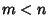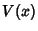## Fractal Sequence

Given an Infinitive Sequencewith associated array, thenis said to be a fractal sequence

1. If, then there existssuch that,

2. If, then, for every, there is exactly onesuch that.
(Asandrange through, the array, called the associative array of, ranges through all of.) An example of a fractal sequence is 1, 1, 1, 1, 2, 1, 2, 1, 3, 2, 1, 3, 2, 1, 3, ....

Ifis a fractal sequence, then the associated array is an Interspersion. Ifis a fractal sequence, then the Upper-Trimmed Subsequence is given by, and the Lower-Trimmed Subsequenceis another fractal sequence. The Signature of an Irrational Number is a fractal sequence.

Kimberling, C. Fractal Sequences and Interspersions.'' Ars Combin. 45, 157-168, 1997.